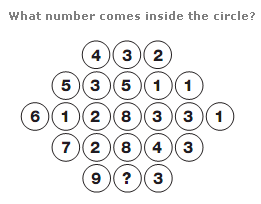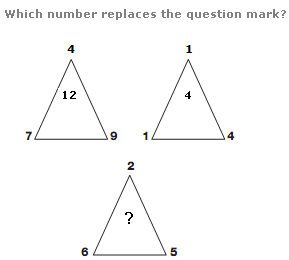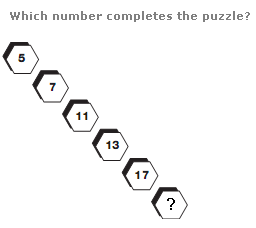# Puzzles - Number puzzles

Exercise : Number puzzles
1.:
6
Explanation
:
Looking at the diagram in rows, the central circle equals half the sum of the numbers in the other circles to the left and right of the centre.

2.:
9
Explanation
:
The number at the centre of each triangle equals the sum of the lower two numbers minus the top number.

3.